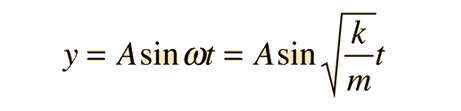# Simple Harmonic Motion(1)

WHAT IS SIMPLE HARMONIC MOTION (SHM)?

Simple harmonic motion is the motion of a mass on a spring when it is subject to the linear elastic restoring force given by Hooke’s Law. The motion is sinusoidal in time and demonstrates a single resonant frequency.

KEY POINT: *The vibration of a guitar string is an example of simple harmonic motion*

When a musician strums a guitar, the vibration of the strings creates sound waves that human ears hear as music. When a guitar string is plucked, it moves a certain distance, depending on how hard the guitar player strums. The string returns to its starting point and travels nearly the same distance in the opposite direction. The vibrational energy of the string is dissipated in the form of sound. This causes the distance the string moves, or the amplitude of the vibrations, to decrease gradually. The volume of the sound fades until the string eventually falls silent.

SIMPLE HARMONIC EQUATIONThe motion equation for simple harmonic motion contains a complete description of the motion, and other parameters of the motion can be calculated from it.The total energy for an undamped oscillator is the sum of its kinetic energy and potential energy, which is constant atSimple harmonic oscillators work because the force acts in the opposite direction to the displacement. As the pendulum moves away from the area immediately below the peg it is hanging on, the force no longer acts in the opposite direction to the displacement.DISPLACEMENT, VELOCITY AND ACCELERATIONThe displacement of a simple harmonic oscillator is:Velocity  is the rate of change of displacement, so:Acceleration is the rate of change of velocity, so:TIME PERIOD

The time period of an oscillation is the time taken to repeat the pattern of motion once. In general:However, depending on the type of oscillation, the value of ω (angular velocity) changes. For a mass on a spring:For a pendulum:ANGULAR VELOCITY

Angular velocity in circular motion is the rate of change of angle. It is measured in radians per. second. Since 2π radians is equivalent to one complete rotation in time period T: